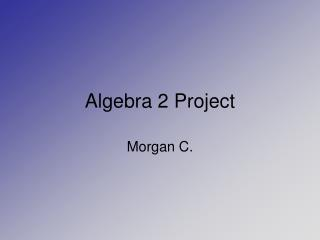DownloadDownload PresentationAlgebra 2 Project

Algebra 2 Project

Télécharger la présentationAlgebra 2 Project

- - - - - - - - - - - - - - - - - - - - - - - - - - - E N D - - - - - - - - - - - - - - - - - - - - - - - - - - -
Presentation Transcript

1. Algebra 2 Project Morgan C.

2. Advanced/Applied Technology • Electronics • I am going to do a problem where I will be trying to find the Current of the circuit.

3. Problem • There is a series circuit with a voltage of 16V and a resistance of 0.050khz. What is the current of this series circuit? • Formula: I=V/R • I is current, V is voltage, R is resistance

4. Solution • I=V/R • I=16V/.05khz • I=320mA

5. Any Questions